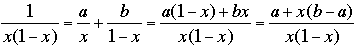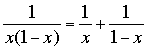SEARCH HOMEMath Central Quandaries & QueriesQuestion from Matt, a teacher: derivative of dx/(x(1-x)) From what I've seen I should break apart the equation as such derivative of dx/x - dx/(1-x) and then get the 2 corresponding log functions. If that is correct why does this factoring work, if that is incorrect what is the proper way to find the derivative. Thanks in advance!Matt,

I think what you want to find is the antiderivative or sometimes called the integral of 1/(x(1 - x)), not the derivative. I would do exactly what you did, write 1/(x(1 - x)) = 1/x + 1/(1 - x) and find the antiderivative of 1/x and 1/(1 - x) separately.

Integration is somewhat of an art that relies on your experience and knowledge of what functions you can already integrate. When I see 1/(x(1 - x)) i recognize that I can integrate a constant divided by x and also a constant divided by (1 - x) so I wonder "Can I write 1/[x(1 - x)] = a/x + b/(1 - x) for some constants a and b?"

Suppose

1/[(x(1 - x)] = a/x + b/(1 - x)

Find a common denominator on the right and add the fractionsIf these fractions are identical then the numerators must be identical as polynomials so

1 = a + x(b - a)

and thus a = 1 and b - a = 0 so a = b = 1 and henceI hope this helps,
HarleyMath Central is supported by the University of Regina and The Pacific Institute for the Mathematical Sciences.